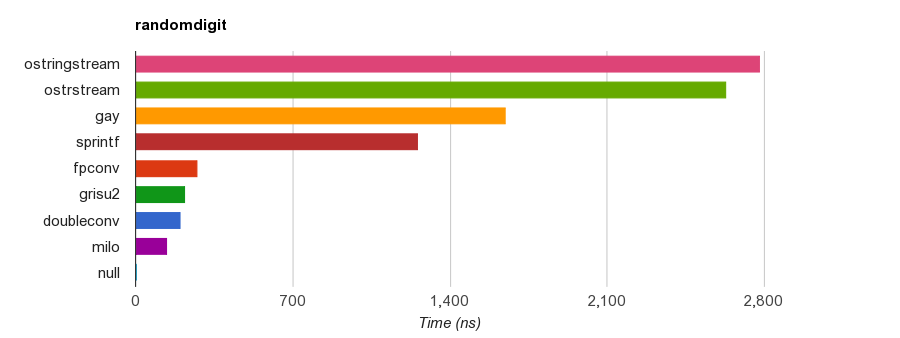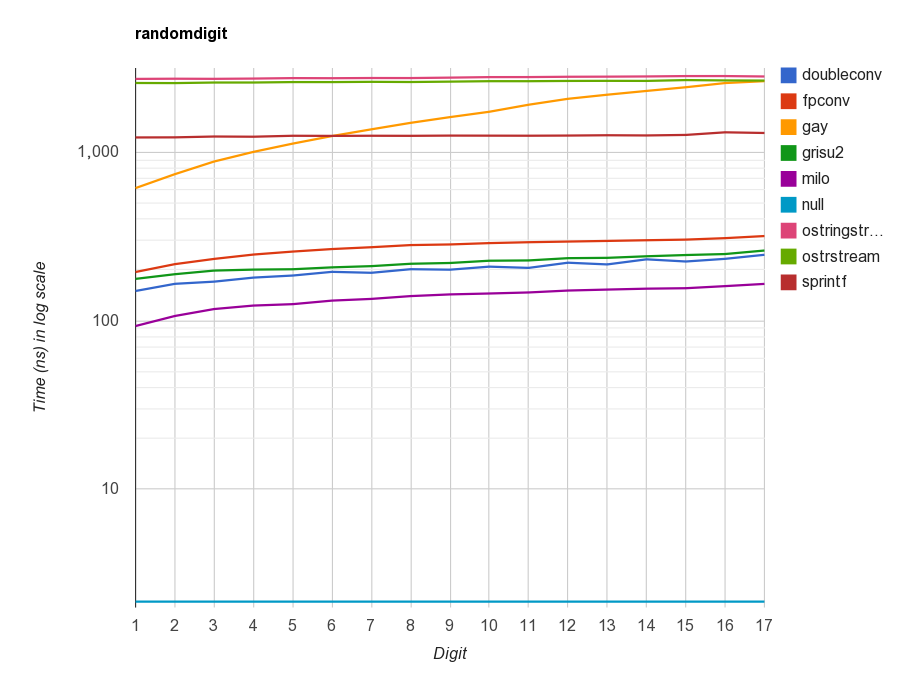# RapidJSON 代码剖析（四）：优化 Grisu

## Grisu是什么

Grisu 是把浮点数转换为字符串的算法。在 Chrome 里执行这段 JavaScript 实际上就调用了 Grisu：

document.write(1/3); // 0.3333333333333333


// 除 +/-inf 及 NaN 外的浮点数都应该传回 true。
bool Verify(double d) {
assert(!isnan(d) && !isinf(d));
char buffer; // 足够大么？
dtoa(buffer, d); // 双精度浮点数转换至字符串，是double-to-ascii
char* end;
double d2 = strtod(buffer, &end); // 字符串转换至双精度浮点数
return *end == '\0' && d == d2;
}


Grisu 的算法非常简单，但它有一个缺点，就是其结果并不像是给人看的。如文中的例子，Grisu 把 0.3 的打印成 29999999999999998e-17。这是「正确的」转换结果，它可以通过 Verify() 验证。## 整数除法优化

uint32_t p1 = /*...*/;
int kappa = 10;
uint32_t div = 1000000000;
while (kappa > 0) {
d = p1 / div; // 第一个除法
if (d || *len)
buffer[(*len)++] = static_cast<char>('0' + static_cast<char>(d));
p1 %= div;
kappa--;
div /= 10;    // 第二个除法
// ...
}


mov    esi, 0xcccccccd  ; 3435973837
imul   rsi, rax
shr    rsi, 0x23        ; 35


while (kappa > 0) {
uint32_t d = 0;
switch (kappa) {
case  9: d = p1 /  100000000; p1 %=  100000000; break;
case  8: d = p1 /   10000000; p1 %=   10000000; break;
case  7: d = p1 /    1000000; p1 %=    1000000; break;
case  6: d = p1 /     100000; p1 %=     100000; break;
case  5: d = p1 /      10000; p1 %=      10000; break;
case  4: d = p1 /       1000; p1 %=       1000; break;
case  3: d = p1 /        100; p1 %=        100; break;
case  2: d = p1 /         10; p1 %=         10; break;
case  1: d = p1;              p1 =           0; break;
default:;
}
if (d || *len)
buffer[(*len)++] = static_cast<char>('0' + static_cast<char>(d));
kappa--;
// ...
}


## 底层优化

struct DiyFp {
DiyFp operator*(const DiyFp& rhs) const {
const uint64_t M32 = 0xFFFFFFFF;
const uint64_t a = f >> 32;
const uint64_t b = f & M32;
const uint64_t c = rhs.f >> 32;
const uint64_t d = rhs.f & M32;
const uint64_t ac = a * c;
const uint64_t bc = b * c;
const uint64_t ad = a * d;
const uint64_t bd = b * d;
uint64_t tmp = (bd >> 32) + (ad & M32) + (bc & M32);
tmp += 1U << 31;  /// mult_round
return DiyFp(ac + (ad >> 32) + (bc >> 32) + (tmp >> 32), e + rhs.e + 64);
}
// ...
uint64_t f;
int e;
};


    DiyFp operator*(const DiyFp& rhs) const {
#if defined(_MSC_VER) && defined(_M_AMD64)
uint64_t h;
uint64_t l = _umul128(f, rhs.f, &h);
if (l & (uint64_t(1) << 63)) // rounding
h++;
return DiyFp(h, e + rhs.e + 64);
#elif (__GNUC__ > 4 || (__GNUC__ == 4 && __GNUC_MINOR__ >= 6)) && defined(__x86_64__)
__extension__ typedef unsigned __int128 uint128;
uint128 p = static_cast<uint128>(f) * static_cast<uint128>(rhs.f);
uint64_t h = static_cast<uint64_t>(p >> 64);
uint64_t l = static_cast<uint64_t>(p);
if (l & (uint64_t(1) << 63)) // rounding
h++;
return DiyFp(h, e + rhs.e + 64);
#else
// 通用实现
#endif
}


    DiyFp Normalize() const {
DiyFp res = *this;
while (!(res.f & (static_cast<uint64_t>(1) << 63))) {
res.f <<= 1;
res.e--;
}
return res;
}


    DiyFp Normalize() const {
#if defined(_MSC_VER) && defined(_M_AMD64)
unsigned long index;
_BitScanReverse64(&index, f);
return DiyFp(f << (63 - index), e - (63 - index));
#elif defined(__GNUC__) && __GNUC__ >= 4
int s = __builtin_clzll(f);
return DiyFp(f << s, e - s);
#else
// 通用实现
#endif
}


## 参考文献

 Coonen, Jerome T. “an Implementation Guide to a Proposed Standard for Floating-Point Arithmetic.” Computer 13.1 (1980): 68-79.

 Steele Jr, Guy L., and Jon L. White. “How to print floating-point numbers accurately.” ACM SIGPLAN Notices. Vol. 25. No. 6. ACM, 1990. http://kurtstephens.com/files/p372-steele.pdf

 Gay, David M. “Correctly rounded binary-decimal and decimal-binary conversions.” Numerical Analysis Manuscript 90-10 (1990). http://ampl.com/REFS/rounding.pdf

 Burger, Robert G., and R. Kent Dybvig. “Printing floating-point numbers quickly and accurately.” ACM SIGPLAN Notices. Vol. 31. No. 5. ACM, 1996. http://www.cs.indiana.edu/~dyb/pubs/FP-Printing-PLDI96.pdf

 Loitsch, Florian. “Printing floating-point numbers quickly and accurately with integers.” ACM Sigplan Notices 45.6 (2010): 233-243. http://www.cs.tufts.edu/~nr/cs257/archive/florian-loitsch/printf.pdf

 Granlund, Torbjörn. “Instruction latencies and throughput for AMD and Intel x86 processors, 2014.” https://gmplib.org/~tege/x86-timing.pdf

 Warren, Henry S. Hacker’s delight. Pearson Education, 2012.